# Filter a Rectangular Pulse with no Ringing

To filter a rectangular pulse without any ringing, there is only one requirement on the filter coefficients:  they must all be positive.  However, if we want the leading and trailing edge of the pulse to be symmetrical, then the coefficients must be symmetrical.  What we are describing is basically a window function.

Consider a rectangular pulse 32 samples long with fs = 1 kHz.  Here is the Matlab code to generate the pulse:

```N= 64;
fs= 1000; % Hz sample frequency
n= 0:N-1; % time index
t= n/fs*1000; % ms
% 1. pulse
x= [0 0 0 0 ones(1,32) zeros(1,28)];
plot(t,x),grid
```

Let’s filter the pulse using a Hanning window.  Note this is not the typical way window functions are used:  here, the window is shorter than the signal vector and we are convolving the window with the signal (not performing an element-by-element multiplication).  Also, we divide the window coefficients by their sum to make the dc component = 1.

```b= hanning(9)/5; % N = 9 Hanning window
stem(b),grid,figure
y= filter(b,1,x); % filter the pulse x
plot(t,y,'o-'),grid
```

The Pulse is shown in Figure 1, the Hanning Window in Figure 2, and the filtered pulse is shown in Figure 3.  The edges of the pulse now have duration equal to the length of the window.  If we use an N= 15 Hanning window, we get slower edges, as shown in Figure 4.

We can compute the spectra of the original pulse and the filtered pulse as follows:

```[h,f]= freqz(x/32,1,512,fs); % spectrum of unfiltered pulse
H= 20*log10(abs(h));
[h,f]= freqz(y/32,1,512,fs); % spectrum of filtered pulse
H_filt= 20*log10(abs(h));
plot(f,H_filt,f,H),grid
```

The pulse spectra for the N= 9 and N= 15 Hanning window cases are shown in Figures 5 and 6.  You can see that the pulse with the faster edges has a wider spectrum.

Finally, we note that these filters are not meant for channel filtering of signals like QAM.  QAM systems typically use square-root Nyquist filters (also called Root Raised Cosine filters), which produce spectrally-efficient pulses with precise spacing of the time-response nulls, in order to minimize inter-symbol interference.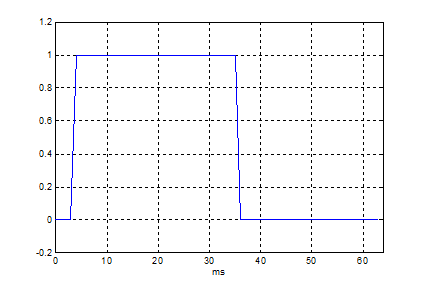Figure 1.  Pulse with duration of 32 samples, fs = 1 kHz.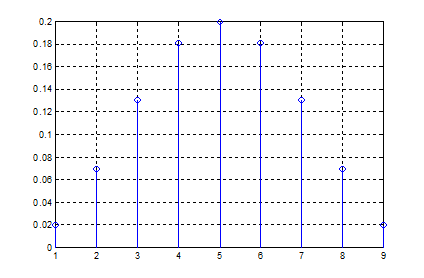Figure 2.  N = 9 Hanning window        hanning(9)/5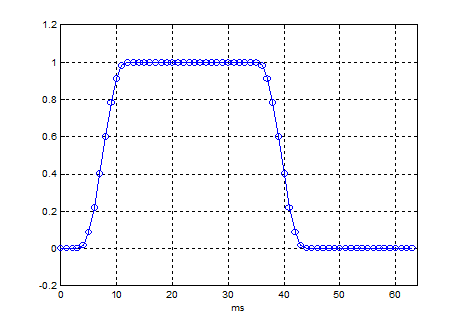Figure 3.  Pulse filtered by N = 9 Hanning window.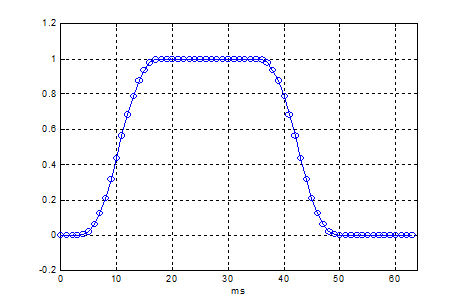Figure 4.  .  Pulse filtered by N = 15 Hanning window.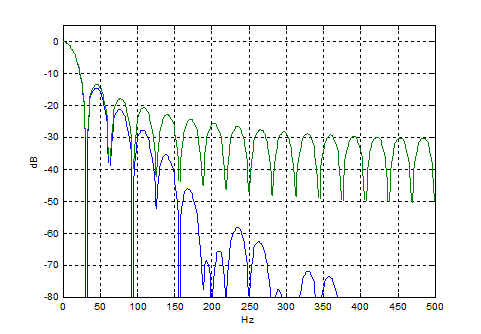Figure 5.  Pulse Spectra.  Blue = Pulse filtered by N = 9 Hanning window

Green = Unfiltered pulse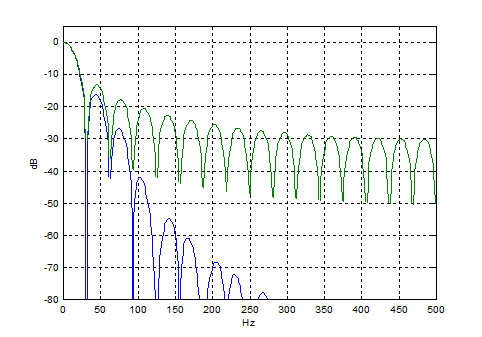Figure 6.  Blue = Pulse filtered by N= 15 Hanning window.

Green = Unfiltered Pulse

m-file

```% pulse_filter1.m 5/12/16 nr
% Filter a rectangular pulse with a hanning window
N= 64;
fs= 1000; % Hz sample frequency
n= 0:N-1; % time index
t= n/fs*1000; % ms
% 1. pulse
x= [0 0 0 0 ones(1,32) zeros(1,28)];
plot(t,x),grid
axis([0 N -.2 1.2]),xlabel('ms'),figure
% 2. filter pulse x with a window function
% edge of filtered pulse has duration = window length
b= hanning(9)/5; % hanning window
%b= hanning(15)/8;
stem(b),grid,figure
y= filter(b,1,x); % filter the pulse x
plot(t,y,'o-'),grid
axis([0 N -.2 1.2]),xlabel('ms'),figure
% 3. Pulse spectra
[h,f]= freqz(x/32,1,512,fs); % spectrum of unfiltered pulse
H= 20*log10(abs(h));
[h,f]= freqz(y/32,1,512,fs); % spectrum of filtered pulse
H_filt= 20*log10(abs(h));
plot(f,H_filt,f,H),grid
axis([0 fs/2 -80 5]),xlabel('Hz'),ylabel('dB')
```

5/12/16

[ - ]
Comment by May 13, 2016Hi. I think this blog makes a good point, i.e., To (lowpass) filter a rectangular pulse without any ringing, there is only one requirement on the filter coefficients: they must all be positive. A simple moving average filter would also have similar behavior.

[ - ]
Comment by May 12, 2016Thanks Neil for this very useful post...
[ - ]
Comment by May 15, 2016Hi, why not just multiply some scale factor(h) by x (both vectors same length) instead of convolution if your target is smoothing the pulse. Thanks
[ - ]
Comment by May 15, 2016Kaz,
The advantage of a filter (convolution) is that the pulse can be of any length, or there can be multiple pulses. Filtering will smooth every edge.
[ - ]
Comment by May 15, 2016Thanks Neil. But I doubt such window will work for all cases, try a train of pulses and see. This is because the frequency domain has to be looked after and cut off point carefully selected.
[ - ]
Comment by May 15, 2016Zac,
I beg to differ! Change 2 lines in the m-file as follows:
original code:
N = 64;
x= [0 0 0 0 ones(1,32) zeros(1,28)];

new code:
N= 128;
x= [0 0 0 0 ones(1,32) zeros(1,28) ones(1,32) zeros(1,32)];

You will find the filtering works great.
regards,
Neil
[ - ]
Comment by May 15, 2016Oops, sorry, I meant Kaz, not Zac!
[ - ]
Comment by May 15, 2016well try this:

x = zeros(1,100);
x(4:4:end) = 1;
x(5:4:end) = 1;
h=hanning(9)

y=filter(h,1,x);
plot(y)
[ - ]
Comment by May 15, 2016Kaz,

OK. I see your problem. For the window to work as a LPF, the pulse should be twice as long as the length of the window, or longer.

Note your pulse train cannot easily be filtered because there are only 2 samples during the pulse duration. In my example, there are 32 samples in the pulse duration.
[ - ]
Comment by May 15, 2016Kaz,

I need to correct myself. Actually, the pulse can be equal to the length of the window or longer (not twice the length of the window).
regards, neil

To post reply to a comment, click on the 'reply' button attached to each comment. To post a new comment (not a reply to a comment) check out the 'Write a Comment' tab at the top of the comments.